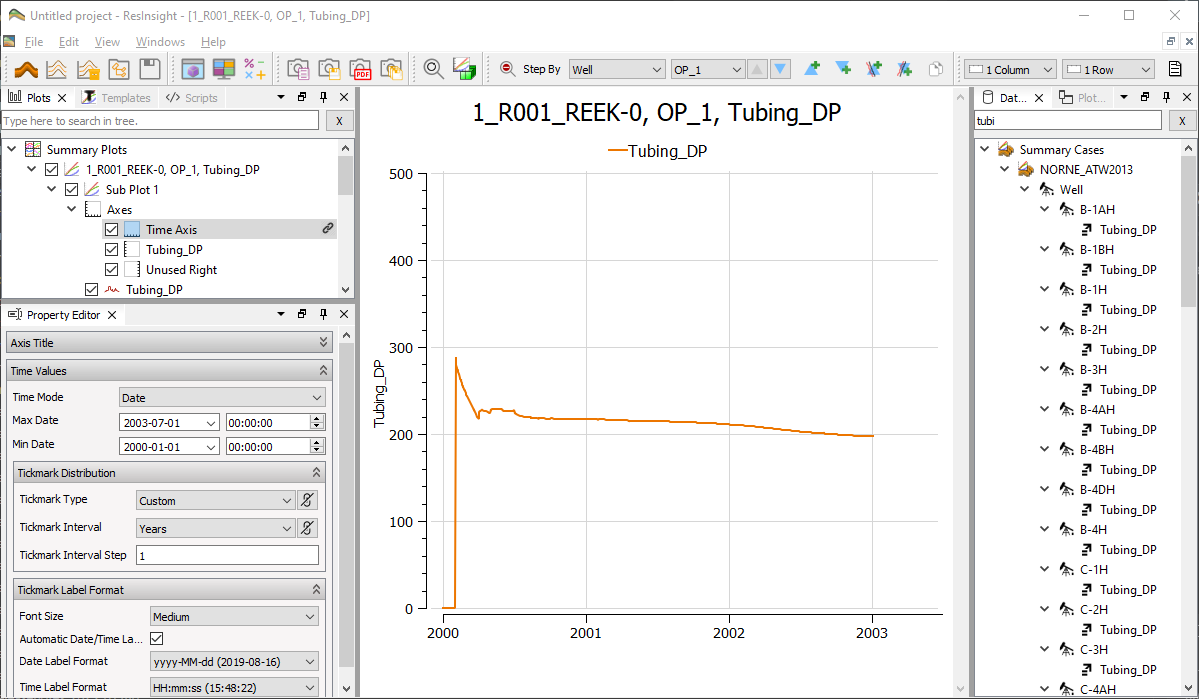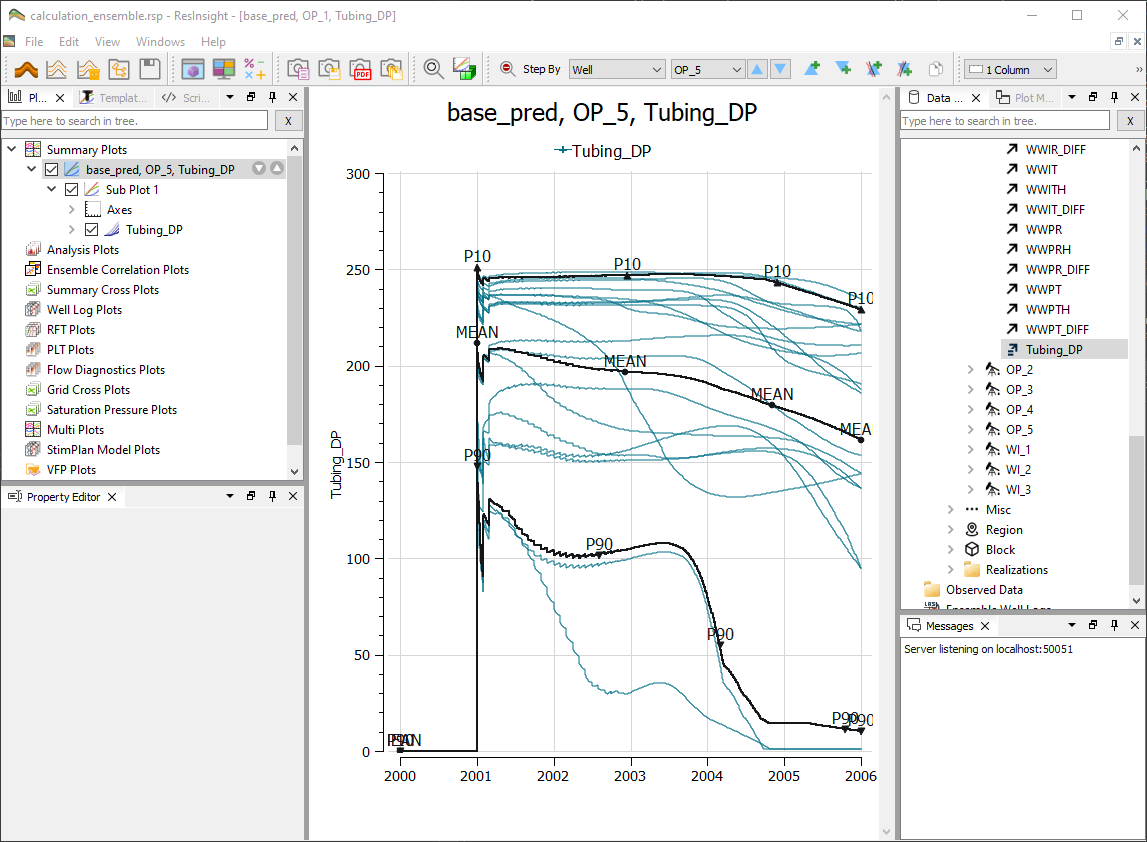# Curve CalculatorThe summary curve calculator is a tool to do calculations on a set of curves. The created curves can be stored for later use in the project.

The calculator can be run by pressing the calculator iconin the menu bar, or by right-clicking on either a summary case or the summary plot collection.

More details on mathematical operations are described in Calculator Expressions

## Calculation Settings

To make a new calculated curve, click on New Calculation. This will add a new calculation to Calculated Summaries. Before choosing which curves to do calculations on, a calculation expression must be made. The default expression Calculation_1 := x + y will do a vector addition on the curves which x and y are placeholders for, and assign it to the calculation Calculation_1.

To assign a summary address to a variable, select a summary vector in Data Sources, and drag/drop this vector into the address field in the Curve Calculator. Further details on variable assignment is covered in section Summary Address Selection.

By default, a calculation defined for one well will be distributed to all other wells. This feature can be disabled by unticking Distribute to other items

### Unit

It is possible to add a unit to the calculated curve, in the field Unit beneath the expression field. This will be used as the label on the y-axis when the curve is displayed in plots.

An expression consists of placeholders (variables) for curves (summary address). By clicking Parse Expression, the variables will appear in the table below the settings. To assign a summary address to a variable, select a summary vector in Data Sources, and drag/drop this vector into the address field in the Curve Calculator.

It is also possible to select the address by pressing the Edit button. This action will open a Summary Address Selection dialog. Use the dialog to select a summary address and press OK.

## Generating CurvesAfter assigning summary addresses to all variables, click Calculate to evaluate the expression. The curve is saved and can be accessed in the Plot Editor.

## Calculation for EnsemblesAll calculation expressions are available for ensemble plotting. Use the calculation objects the same way as they are used for single summary cases.

The similar concept is also used for Grid Property Calculator